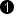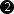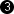## Operator InsertLine

The operator InsertLine multiplexes the lines of N input links I .. I[n-1] into the output link O. Thus the operator outputs the input image lines of all inputs in sequential order at O.

The operator forwards the input image lines to the output one after the other. With every new frame the operator starts with input I. First, the first line of input I is processed and forwarded to the output. Next, the first line of input I is processed. After the first line of I[n-1] has been processed, the operator continuous with the second line of I etc. The operator waits until the required line at the currently selected input is present. Therefore it is not possible to skip inputs. There is no "first come first processed" strategy.

As explained, the operator only forwards a specific input at a certain time. While one input is processed all other inputs are blocks. If an image line is present at a blocked input, ensure that it can be buffered until it's processed. Moreover, users have to ensure that multiple inputs are not sourced by the same image source as this will cause a deadlock. Check tutorial 'Multiplex the Images of Two Cameras' for more information on correct inserting. The example is similar for the InserLine operator.

For every input I[k], the operator provides a controlling input Ins[k]. These inputs are used to control whether the data of an image line has to be used and forwarded to the output or should be discarded. The Ins[k] inputs accept binary data. If a one is provided for the very first pixel of a respective image line, the line will be forwarded to the output. Whereas if value zero is provided for the very first pixel of a respective image line, the line will be processed but discarded.

The I[k] and Ins[k] pairs are synchronous i.e. they have to be sources by the same M-type source though an arbitrary network of O-type operators. See 'M-type Operators with Multiple Inputs' for more information. Therefore, no SYNC operator is required for these pairs (different in VA versions < VA2).

The output image size of the operator is n times higher than the input image sizes. However, the output parallelism is not increased. Therefore, the output bandwidth is equal to the input bandwidth while more data might be required to be transferred. It might be necessary to increase the parallelism of the inputs before the operator is processing the data.

All images at I[k] can be of different width. However, the height of all images has to be equal. Control inputs Ins[k] are ignored for empty input lines. These lines will not be forwarded to the output i.e. will be discarded.

### I/O Properties

Property Value
Operator Type M
Input Links I, data input
I[k], data input
Ins[k], control input
Output Link O, data output

### Supported Link Format

Bit Width [1,64]as I 1 as I
Arithmetic {unsigned, signed} as I unsigned as I
Parallelism any as I as I as I
Kernel Columns any as I 1 as I
Kernel Rows any as I 1 as I
Img Protocol {VALT_IMAGE2D, VALT_LINE1D} as I as I as I
Color Format any as I VAF_GRAY as I
Color Flavor any as I FL_NONE as I
Max. Img Width any any as I[k] autoMax. Img Height any as I as I[k] N * IThe range of the input bit width is [1, 64] for unsigned inputs. For signed inputs, the range is [2, 64]. For unsigned color inputs, the range is [3, 63] and for signed color, the range is [6, 63]. The maximum output image width is the maximum of all input image widths. As of VisualApplets version 3.3.0, and only if in the Simulation Build Setttings the option Simulate 1D Line By Line is selected: If the Image Protocol is set to VALT_LINE1D, the maximum output image height is forced to 1.

Synchronous and Asynchronous Inputs

• Synchronous Groups: I[k] and Ins[k]
• All groups are asynchronous to each other.

None

### Examples of Use

The use of operator InsertLine is shown in the following examples: